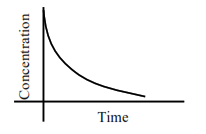# For the following graphs,Question:

For the following graphs,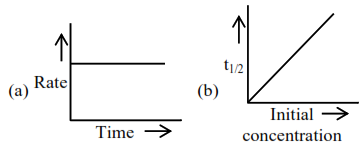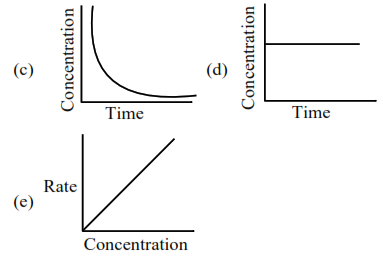Choose from the options given below, the correct one regarding order of reaction is :

1. (b) zero order (c) and (e) First order

2. (a) and (b) Zero order (e) First order

3. (b) and (d) Zero order (e) First order

4. (a) and (b) Zero order (c) and (e) First order

Correct Option: 1

Solution:

For zero order reaction's rate $=\mathrm{K}$ [Reactant] $^{0}$

$\Rightarrow \mathrm{r}=\mathrm{k}$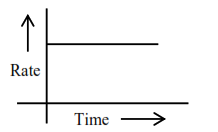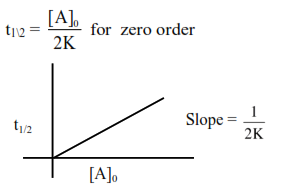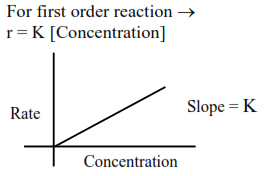Reactant concentration after time $t \rightarrow$

$\mathrm{C}_{\mathrm{t}}=\mathrm{C}_{0} \mathrm{e}^{-\mathrm{kt}}$